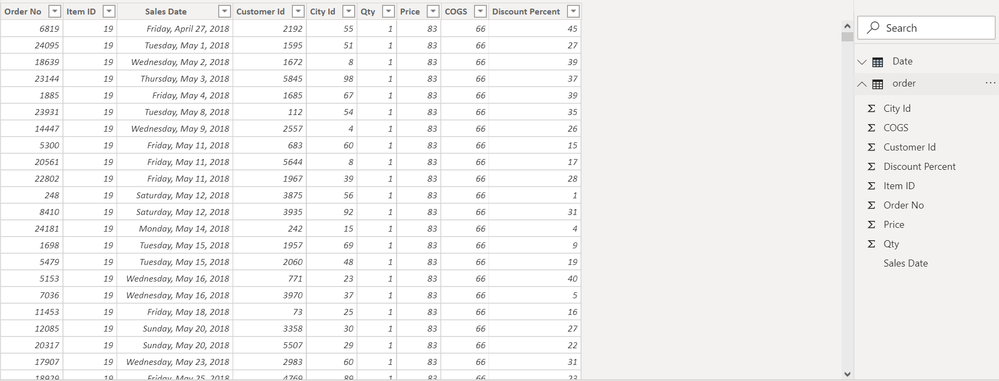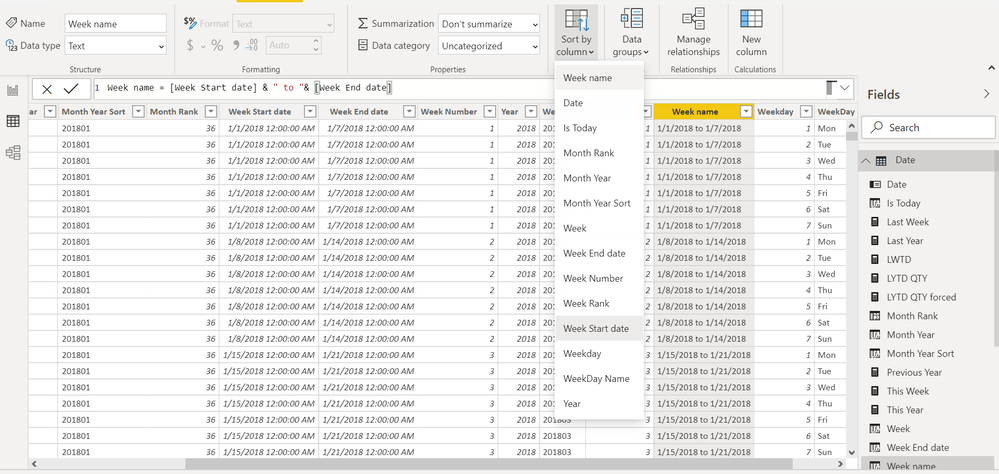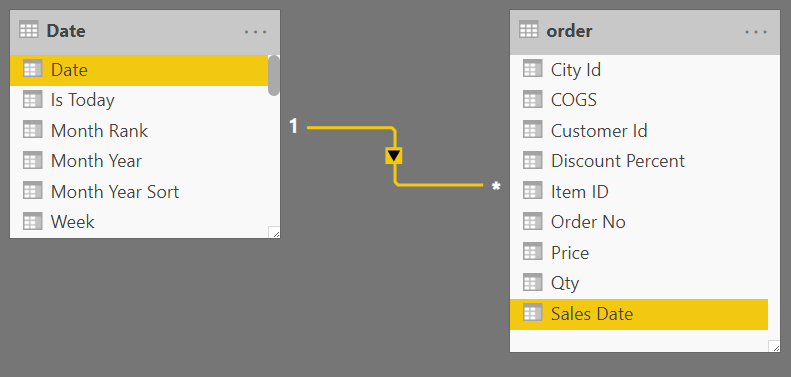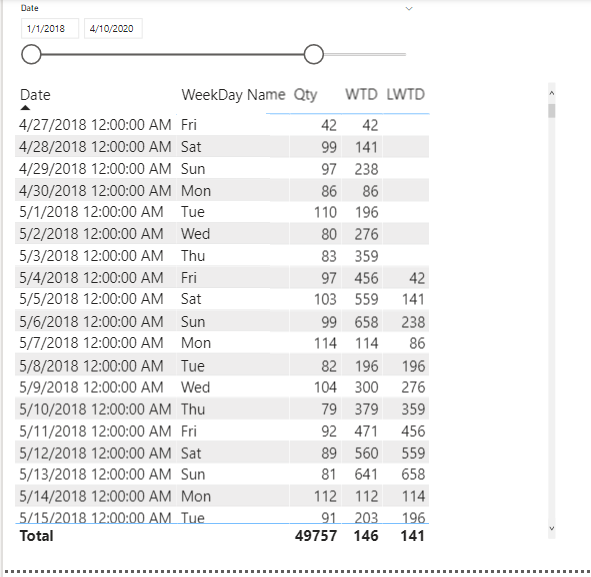cancel
Showing results for
Did you mean:## Week Is Not So Weak: WTD, Last WTD, and This Week vs Last Week

Objective: We would like to have WTD(Week Till Date) Last WTD and This Week vs  Last Week.

Dataset: We have taken Sales data. The data is from April 2018 till April 2020. We have created a date Calendar.Steps: We create a Date Calendar With Monday to Sunday Week, with the following week-related columns.

``````Week Start date = 'Date'[Date]+-1*WEEKDAY('Date'[Date],2)+1
Week End date = 'Date'[Date]+ 7-1*WEEKDAY('Date'[Date],2)
Week Number = WEEKNUM([Date],2)
Week = if('Date'[Week Number]<10,'Date'[Year]*10 & 'Date'[Week Number],'Date'[Year]&'Date'[Week Number])
Week Rank = RANKX(all('Date'),'Date'[Week Start date],,ASC,Dense)
Week name = [Week Start date] & " to "& [Week End date]
Weekday = WEEKDAY([Date],2)
WeekDay Name = FORMAT([Date],"ddd")
``````Marked Date Table as a Date table.

Marked Week Start Date and Sort column for Week NameCreated relation between Date Table and Order TableNow, to have Week Vs Last Week, we will use Week Rank, and the way to go is in the filter in the manner shown below:

FILTER(ALL('Date'),'Date'[Week Rank]=max('Date'[Week Rank])).

``````This Week = CALCULATE(sum('order'[Qty]), FILTER(ALL('Date'),'Date'[Week Rank]=max('Date'[Week Rank])))
Last Week = CALCULATE(sum('order'[Qty]), FILTER(ALL('Date'),'Date'[Week Rank]=max('Date'[Week Rank])-1))``````For WTD, we will also use Weekday.

``````WTD = CALCULATE(sum('order'[Qty]), FILTER(ALL('Date'),'Date'[Week Rank]=max('Date'[Week Rank]) && 'Date'[Weekday] <=max('Date'[Weekday])))
LWTD = CALCULATE(sum('order'[Qty]), FILTER(ALL('Date'),'Date'[Week Rank]=(max('Date'[Week Rank]) -1) && 'Date'[Weekday] <=max('Date'[Weekday])))``````Pbix is attached to this blog.